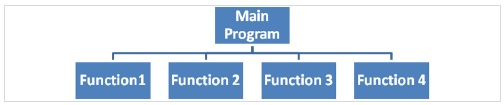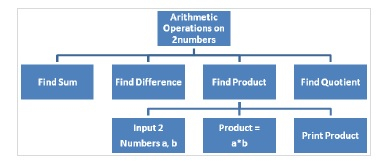# Explain top-down design and structure chart of function in C language

A function is a self-contained block that carries out a specific well defined task.

Advantages of functions in C language include −

• Reusability.
• The length of the program can be reduced.
• It is easy to locate and find any faulty function.
• It facilitates top-down modular programming.

## Top down design and structure charts

It is a problem solving method in which a complex problem is solved by splitting into sub problems.

Structure chart is a documentation tool that shows the relationships among the sub problems of a problem.The splitting of a problem into its related sub problems is the process of refining an algorithm. For example, performing arithmetic operations on 2 numbers, we can do the following −

• Find sum.
• Find difference.
• Find product.
• Find quotient.

Refined algorithm for first step is as follows −

• Take 2 numbers a, b
• Find sum, c = a + b
• Print sum

## Structure chart